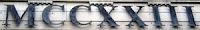## Wednesday, December 17, 2008

### 1223

1223 is a prime number. It is a Sophie Germain prime because 2 x 1223 + 1 = 2447, which is also prime.

1223 is a number that cannot be written as a sum of three squares.

1223 is a prime p such that p + 3 is a semiprime: 1223 + 3 = 1226 = 2 x 613.

1223 is a number n such that n and n + 8 are both primes: 1223 and 1231.

1223 is the smallest number with complexity 24. The complexity of an integer is the least number of 1s needed to represent it using only additions, multiplications, and parantheses.1223 Neckar is an asteroid discovered in 1931.